Question

# Assume that a procedure yields a binomial distribution with a trial repeated n=5 times. Use some...

Assume that a procedure yields a binomial distribution with a trial repeated n=5 times. Use some form of technology like Excel or StatDisk to find the probability distribution given the probability p=0.808 of success on a single trial. k P(X = k) 0 1 2 3 4 5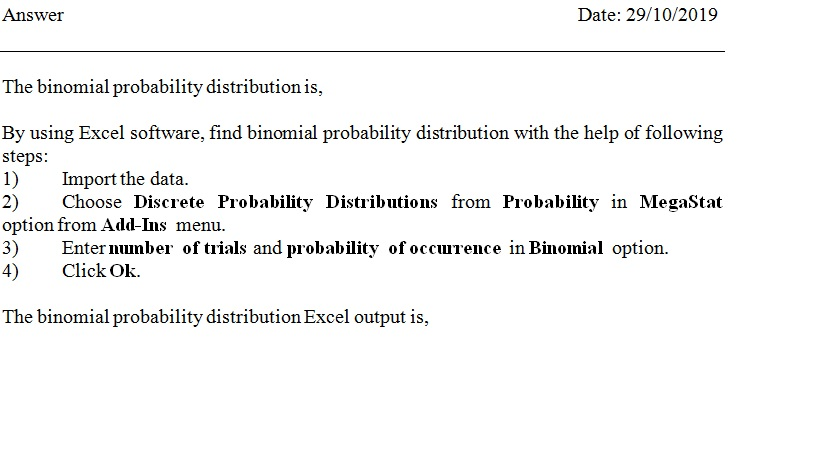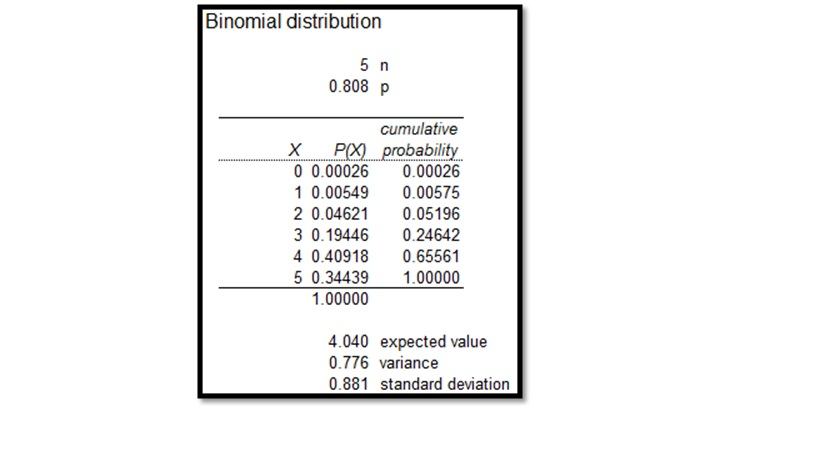#### Earn Coins

Coins can be redeemed for fabulous gifts.

Similar Homework Help Questions
• ### Assume that a procedure yields a binomial distribution with a trial repeated n = 5 times....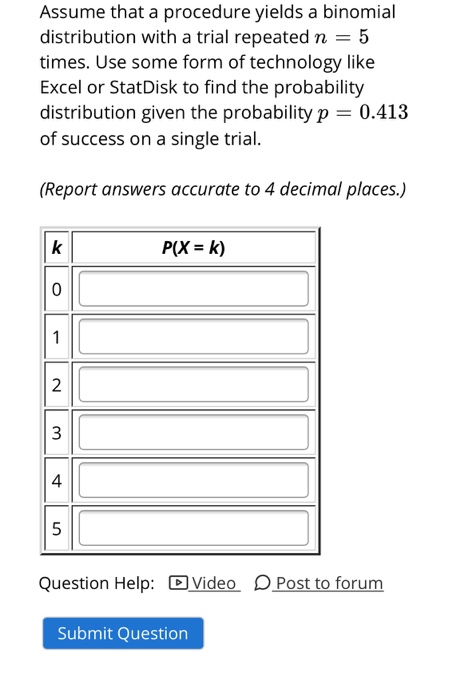Assume that a procedure yields a binomial distribution with a trial repeated n = 5 times. Use some form of technology like Excel or StatDisk to find the probability distribution given the probability p = 0.413 of success on a single trial. (Report answers accurate to 4 decimal places.) k P(X = k) 0 1 N 3 4 5 Question Help: Video Post to forum Submit Question

• ### Assume that a procedure yields a binomial distribution with a trial repeated n = 14 n...

Assume that a procedure yields a binomial distribution with a trial repeated n = 14 n = 14 times. Use either the binomial probability formula (or a technology like Excel or StatDisk) to find the probability of k = 14 k = 14 successes given the probability p = p = 23/30 of success on a single trial. (Report answer accurate to 4 decimal places.) P ( X = k ) =

• ### Assume that a procedure yields a binomial distribution with a trial repeated n = 8 times....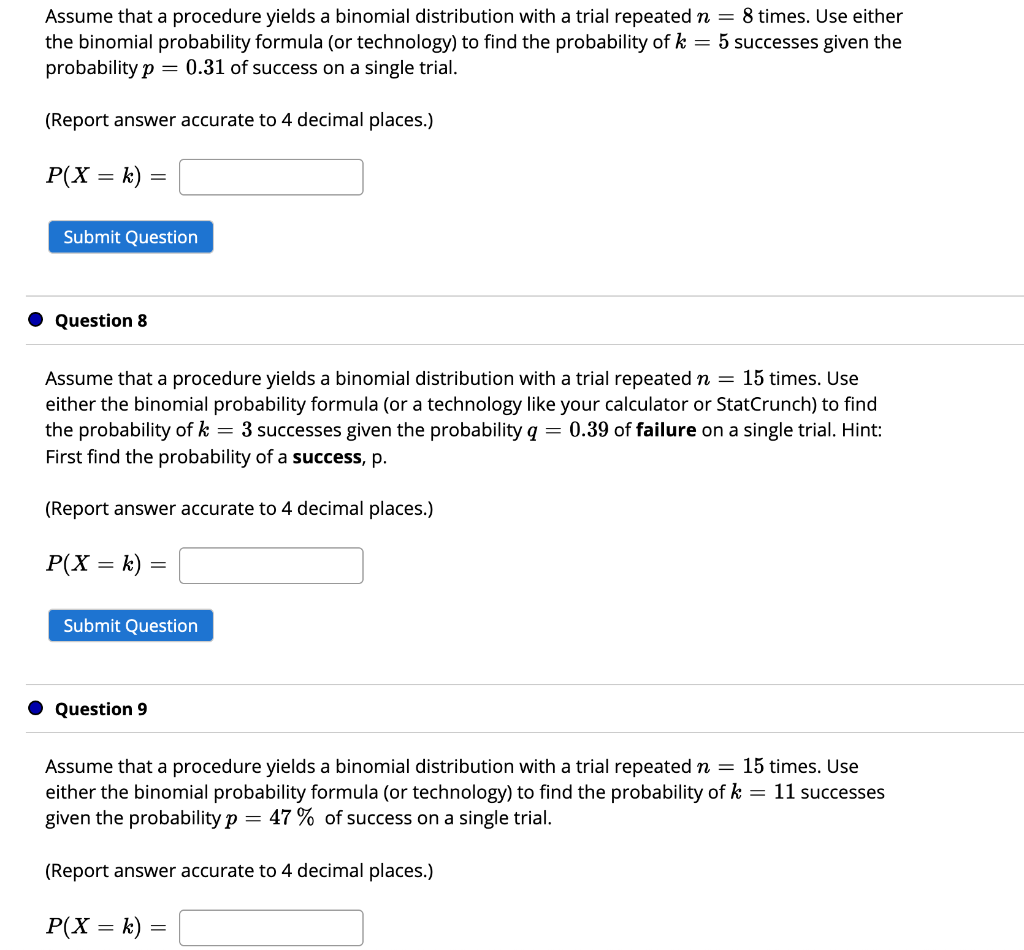Assume that a procedure yields a binomial distribution with a trial repeated n = 8 times. Use either the binomial probability formula (or technology) to find the probability of k = 5 successes given the probability p = 0.31 of success on a single trial. (Report answer accurate to 4 decimal places.) P(X = k) = Submit Question Question 8 Assume that a procedure yields a binomial distribution with a trial repeated n = 15 times. Use either the binomial...

• ### Assume that a procedure yields a binomial distribution with a trial repeated n = 5 times....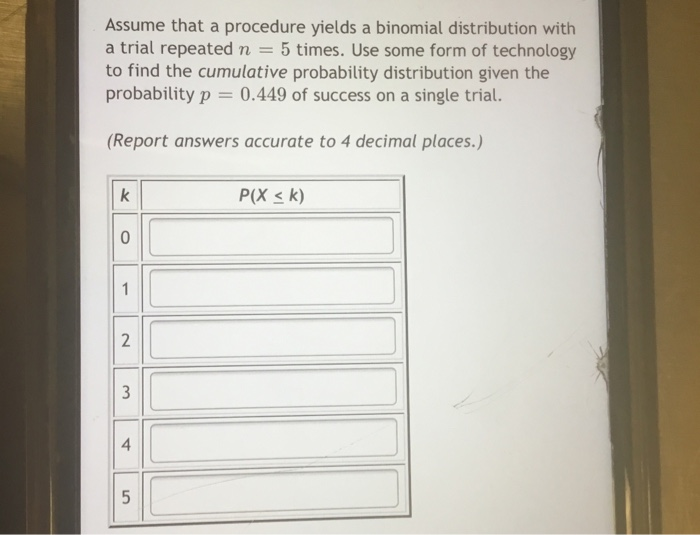Assume that a procedure yields a binomial distribution with a trial repeated n = 5 times. Use some form of technology to find the cumulative probability distribution given the probability p = 0.449 of success on a single trial. (Report answers accurate to 4 decimal places.) k P(X = k) 0 N 3 4 5

• ### Assume that a procedure yields a binomial distribution with a trial repeated n = 5 times....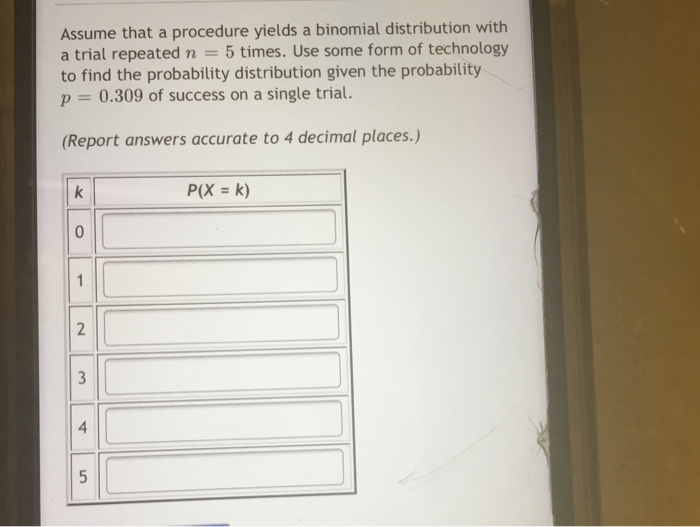Assume that a procedure yields a binomial distribution with a trial repeated n = 5 times. Use some form of technology to find the probability distribution given the probability p = 0.309 of success on a single trial. (Report answers accurate to 4 decimal places.) k P(X = k) 0 1 2 3 4 5

• ### Assume that a procedure yields a binomial distribution with a trial repeated n = 5 times....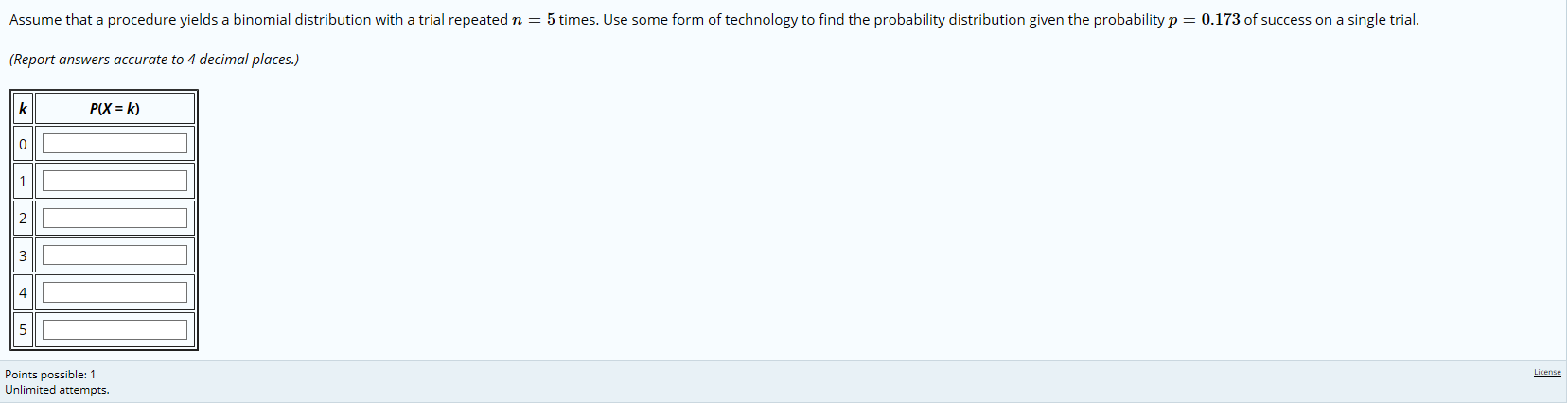Assume that a procedure yields a binomial distribution with a trial repeated n = 5 times. Use some form of technology to find the probability distribution given the probability p= 0.173 of success on a single trial. (Report answers accurate to 4 decimal places.) P(X = k) License Points possible: 1 Unlimited attempts.

• ### Assume that a procedure yields a binomial distribution with a trial repeated n = 5 times....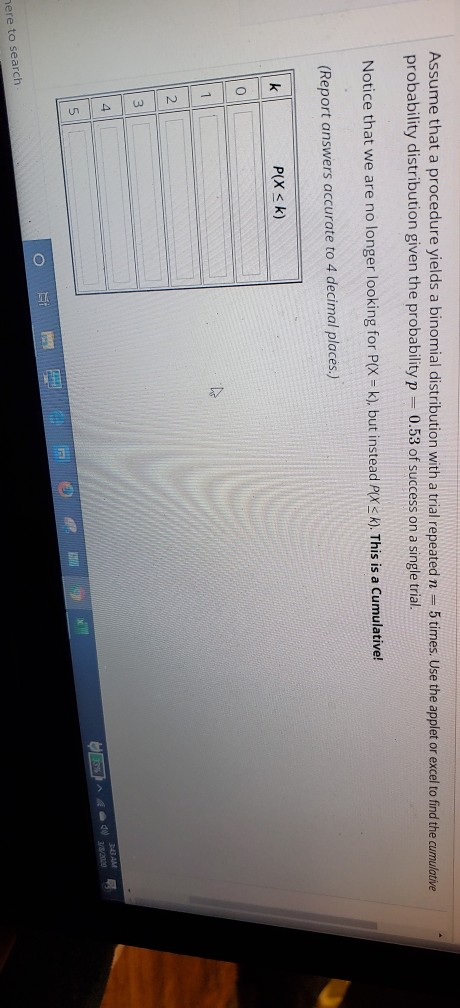Assume that a procedure yields a binomial distribution with a trial repeated n = 5 times. Use the applet or excel to find the cumulative probability distribution given the probability p = 0.53 of success on a single trial. Notice that we are no longer looking for P(X = k), but instead PIX<k). This is a Cumulative! (Report answers accurate to 4 decimal places.) PIX <k) OM 1993 here to search Stats for Analytics:220110447 ... Week 6 Recitation Week 6...

• ### Assume that a procedure yields a binomial distribution with a trial repeated n=5n=5 times. Use some...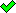Assume that a procedure yields a binomial distribution with a trial repeated n=5n=5 times. Use some form of technology to find the cumulative probability distribution given the probability p=0.155p=0.155 of success on a single trial. (Report answers accurate to 4 decimal places.) k P(X < k) 0 1 2 3 4 5 We were unable to transcribe this imageWe were unable to transcribe this imageWe were unable to transcribe this imageWe were unable to transcribe this imageWe were unable to...

• ### Assume that a procedure yields a binomial distribution with a trial repeated n = 9 times....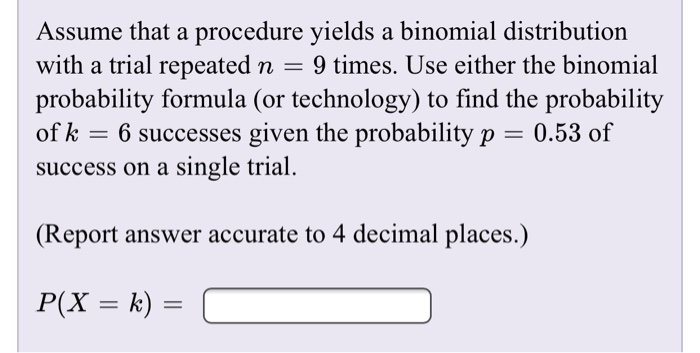Assume that a procedure yields a binomial distribution with a trial repeated n = 9 times. Use either the binomial probability formula (or technology) to find the probability of k = 6 successes given the probability p = 0.53 of success on a single trial. (Report answer accurate to 4 decimal places.) P(X = k) = C

• ### Assume that a procedure yields a binomial distribution with a trial repeated n times. Use the...

Assume that a procedure yields a binomial distribution with a trial repeated n times. Use the binomial probability formula to find the probability of x successes given the probability p of success on a single trial. n=5​, x=2​, p=0.15